Function Repository Resource:

# DirectedAcyclicEvaluate

Evaluate functions locally over any directed acyclic graph

 ResourceFunction["DirectedAcyclicEvaluate"][graph,rules] assigns values to the vertices of a directed acyclic graph by recursively summing over preceding values, starting from initial values listed in rules. ResourceFunction["DirectedAcyclicEvaluate"][graph,rules,vfun] allows for a wide range of calculations via specified function vfun, which evaluates locally on each vertex (see details). ResourceFunction["DirectedAcyclicEvaluate"][expr] allows specification of expr as an Association with the keys "Graph", "VertexWeights", "VertexFunction", and optionally "EdgeWeights".

## Details

Graph vertices can be thought of as named memory locations for both inputs and outputs.
Evaluation distinguishes inputs as the set of root vertices not pointed to by any directed edge (sources).
All other vertices reached by following directed edges are considered outputs.
A vertex vi and its edge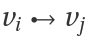are both said to precede another vertex vj, if the graph indeed contains edge.
Values (or weights) wi are assigned to vertices vi using rules of the form viwi.
Input rules should list viwi for all input vertices vi.
If input values are missing from rules, vertex names vi are assumed as values.
If rules includes a rule for an output vertex vi, the associated value is ignored and overwritten upon evaluation.
Function vfun accepts three arguments parallel over index i:
 #1 a list of values wi on all vertices vi preceeding vj #2 a list of edge weights wij for each preceeding edge#3 a list of all preceeding edgesIf vfun is specified as a symbol sym (such as Times or Plus), then vfun=sym@@#1&.
Optional specification of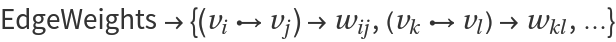may simplify some calculations.
Values in #2 are obtained most efficiently using an Association, so it is possible to get a Missing["KeyAbsent",_] error if the list of EdgeWeights is incomplete.
ResourceFunction["DirectedAcyclicEvaluate"] also acts as Evaluate on an Unevaluated expr, which is formatted as an Association.
Association expr has a primary key "Graph", which should point to a directed acyclic graph.
Optional Keys of the Association and their default values are:
 "VertexWeights" {} a list of function values "VertexFunction" Plus determines how to fold over incoming values "EdgeWeights" {} weights or phases per graph edge
The Association expr is then effectively its own type of expression, which should have a definite evaluation.
Any expr that conforms to the schema outlined above should ultimately be easy to compile for optimized evaluation.
Ultimately, ResourceFunction["DirectedAcyclicEvaluate"] returns a complete Association listing all function values under key "VertexWeights".
The output Association is certified in the sense that it is locally checkable on every graph vertex.

## Examples

### Basic Examples (3)

Enumerate the first few terms of the Fibonacci sequence:

 In:=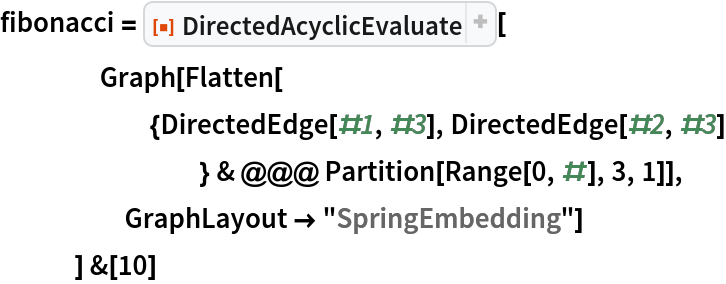Out=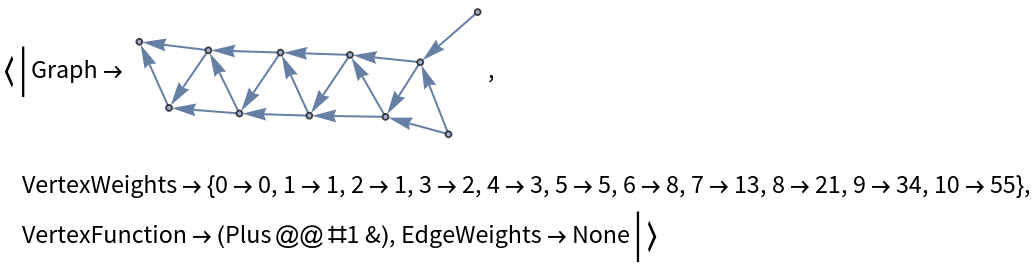Compare with pre-compiled function Fibonacci:

 In:=Out=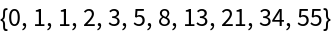Depict the Fibonacci calculation on its directed graph:

 In:=Out=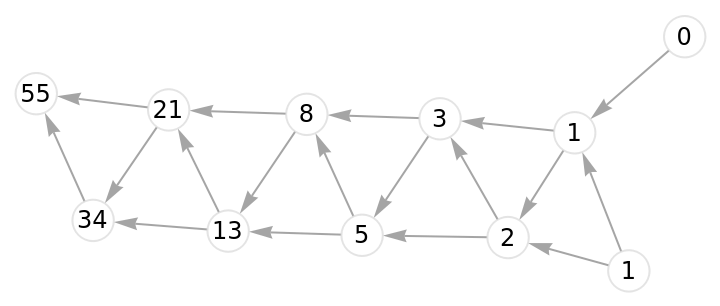### Scope (5)

Count walks on a directed grid:

 In:=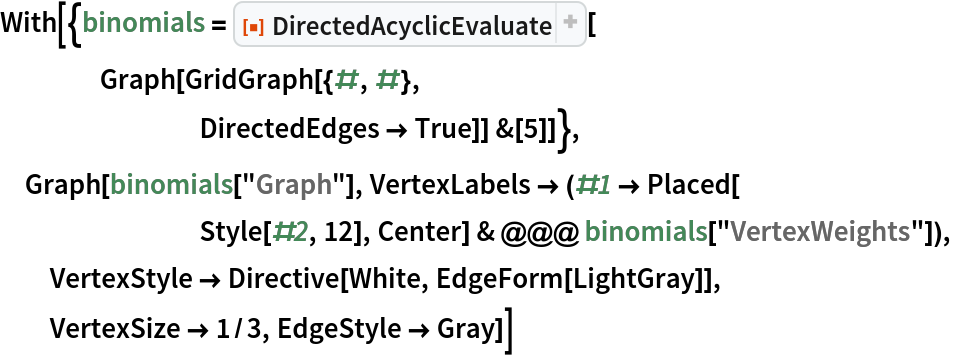Out=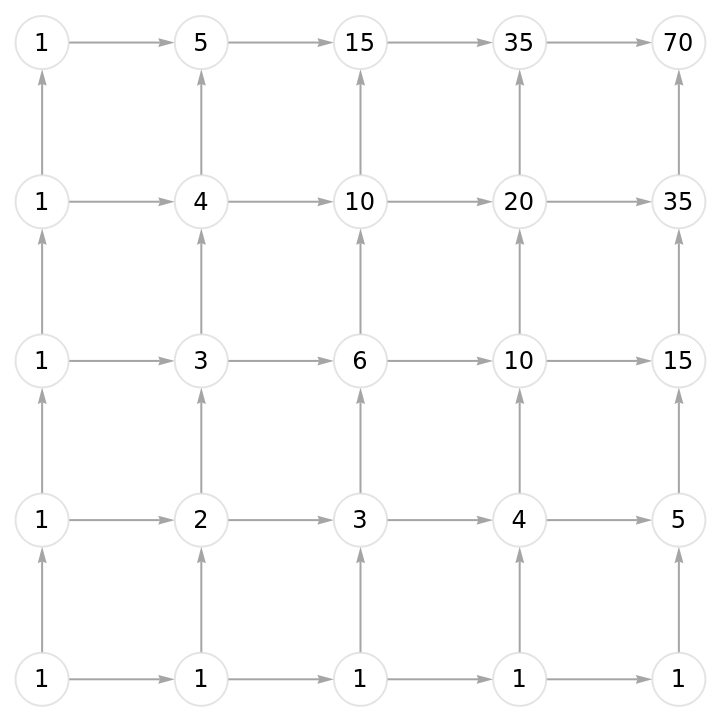Convert to partial probabilities by adding edge weights:

 In:=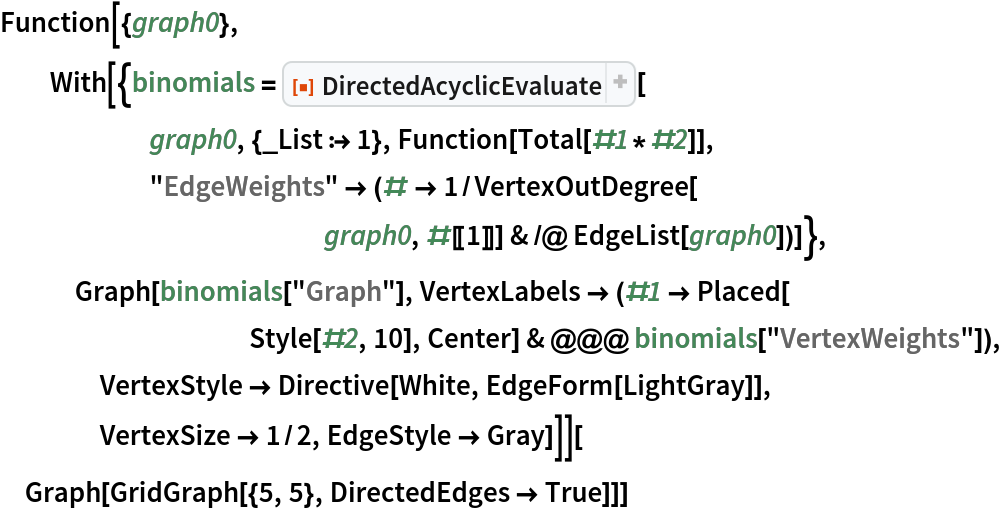Out=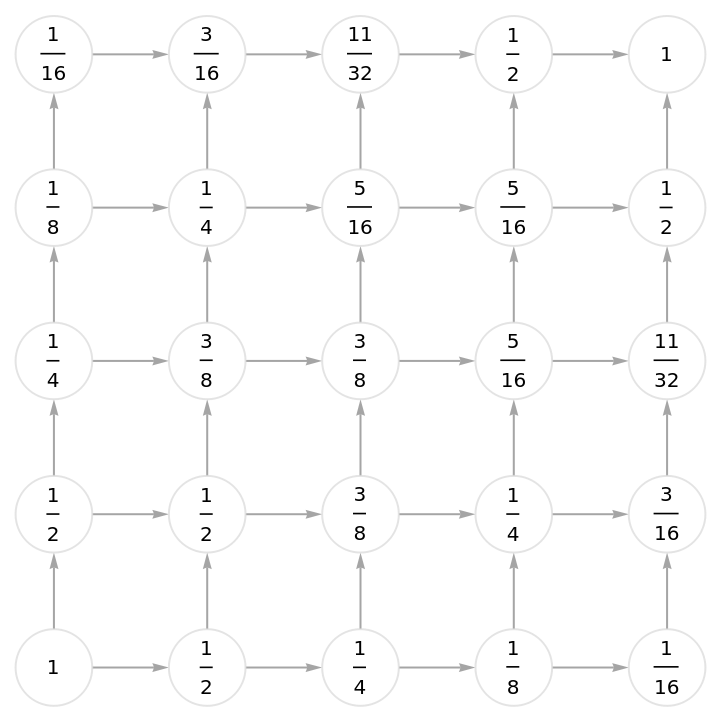Count the leaves on a random tree:

 In:=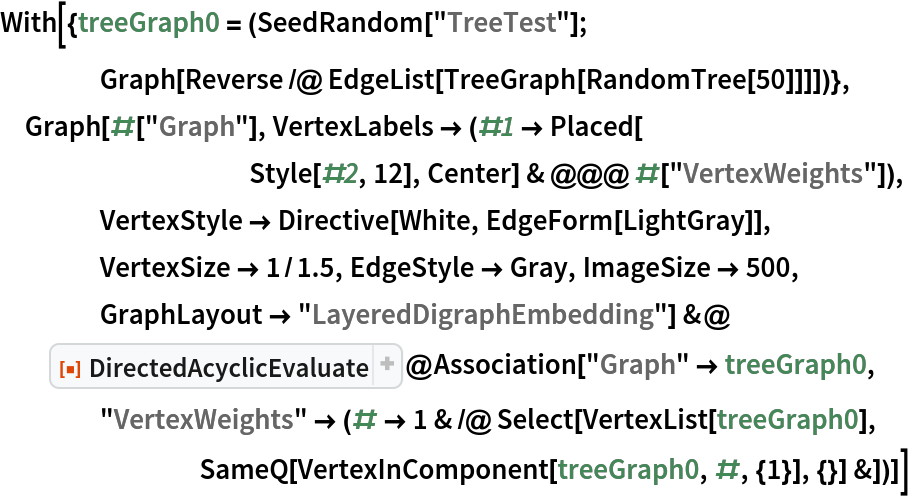Out=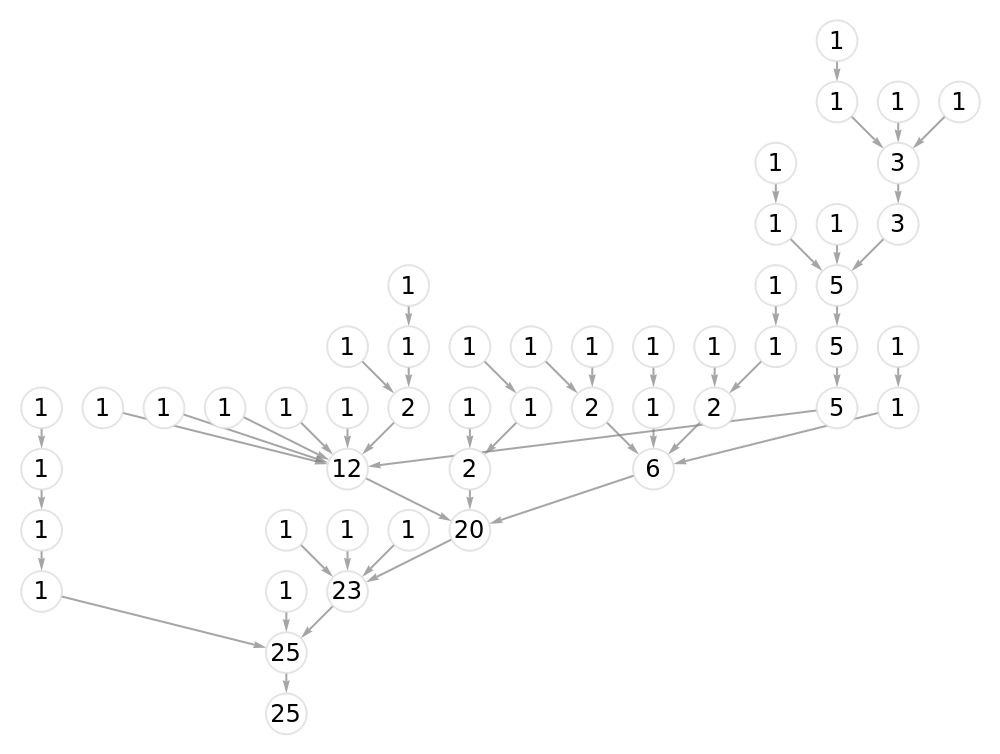Evaluate a Factorial number using binary splitting:

 In:=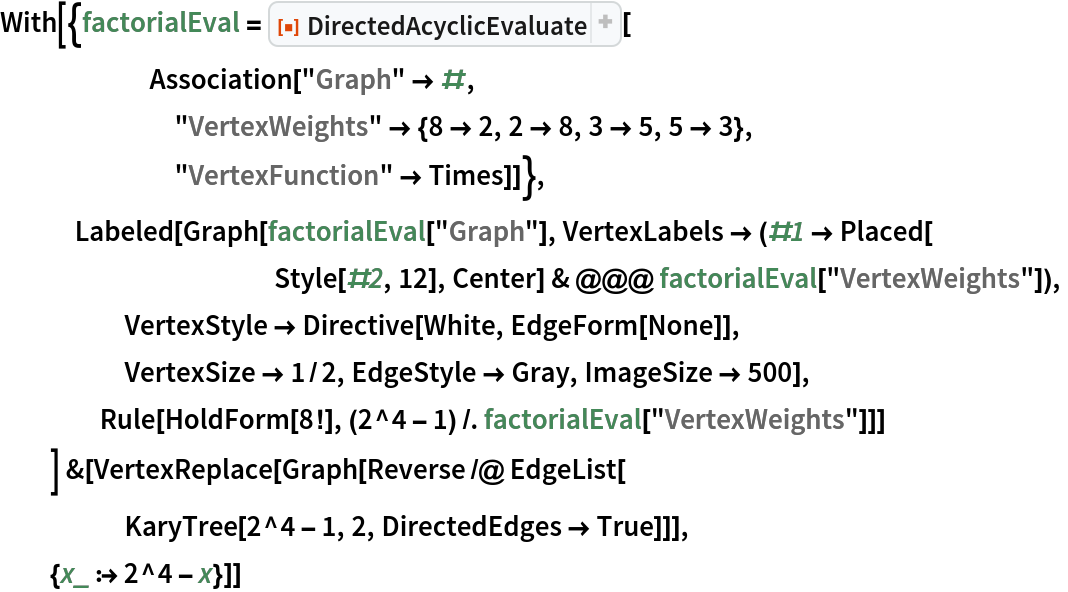Out=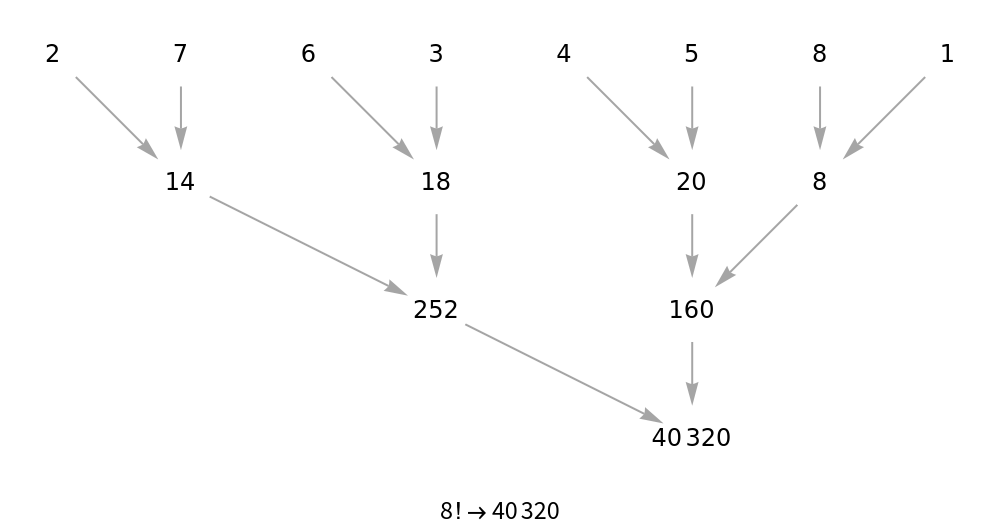Use symbolic functions to create an encoding of 24 branching and merging traversals of a hypercube:

 In:=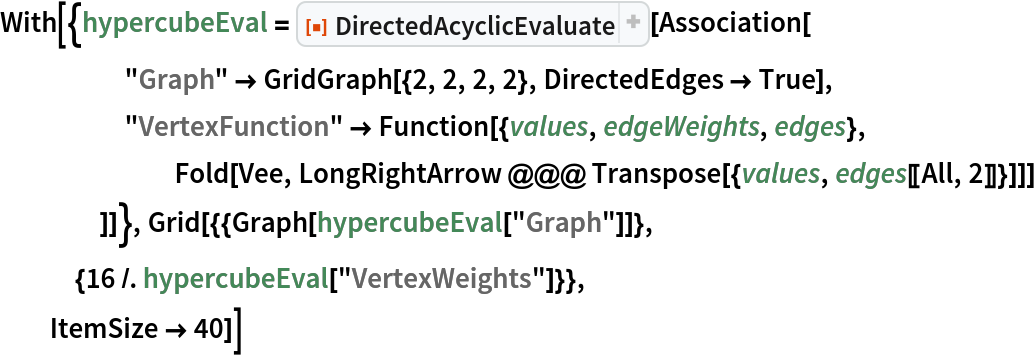Out=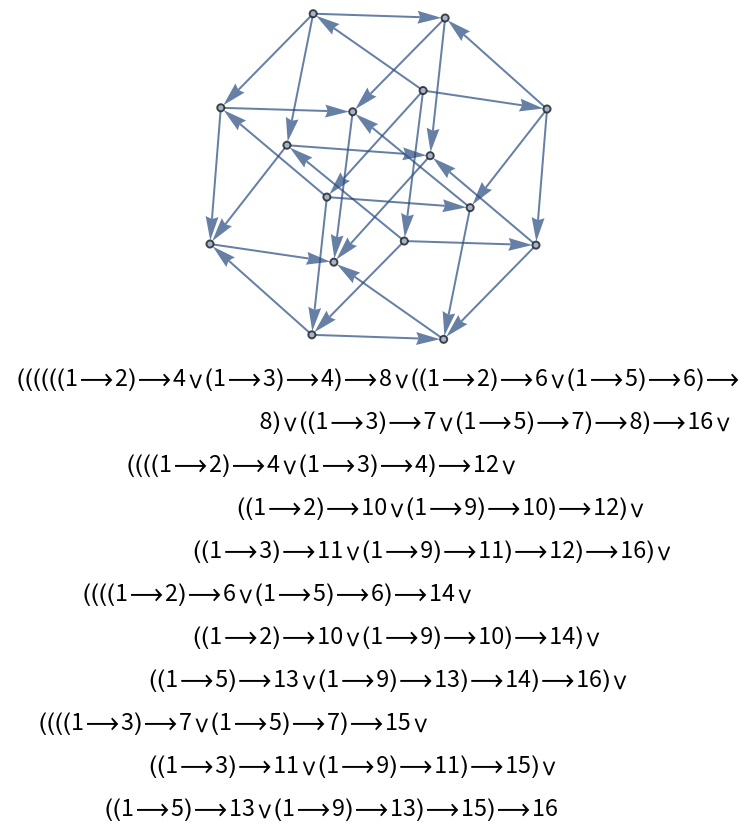Split path symbols based on parity of the outgoing and incoming vertices:

 In:=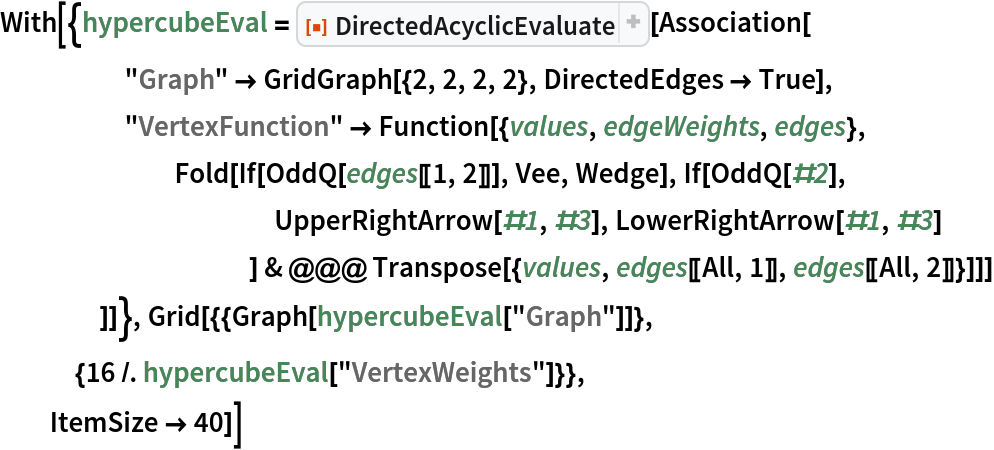Out=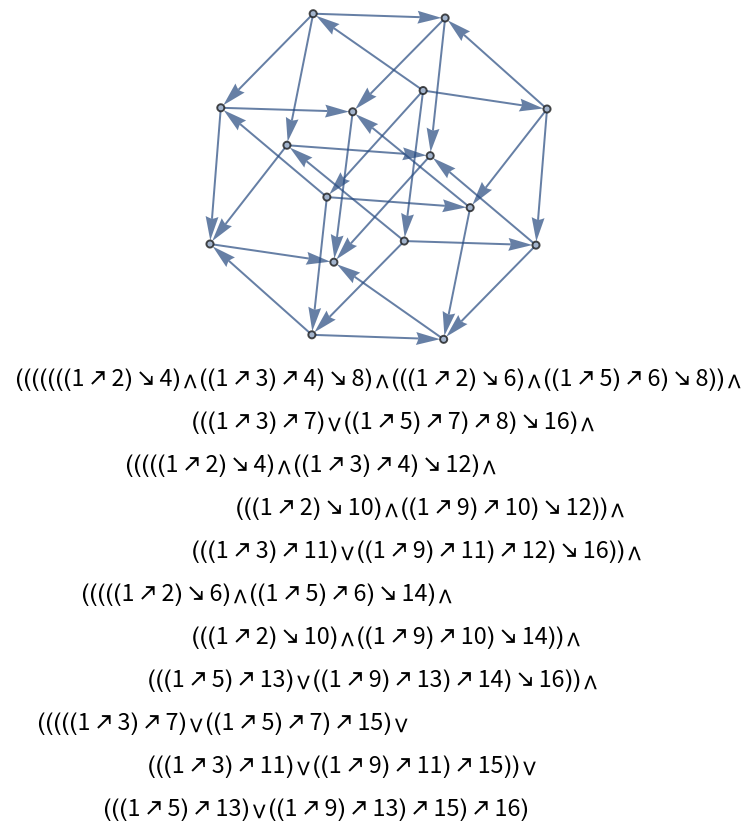### Properties and Relations (2)

Valid output Associations should cause the following check function to list zeroes:

 In:=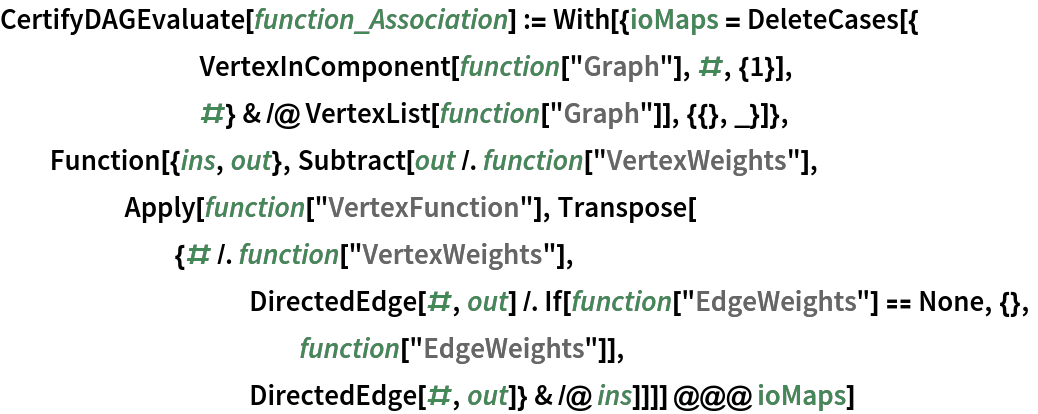Double check a p-recurrence important to the theory of algebraic sphere curves (cf. OEIS A318495):

 In:=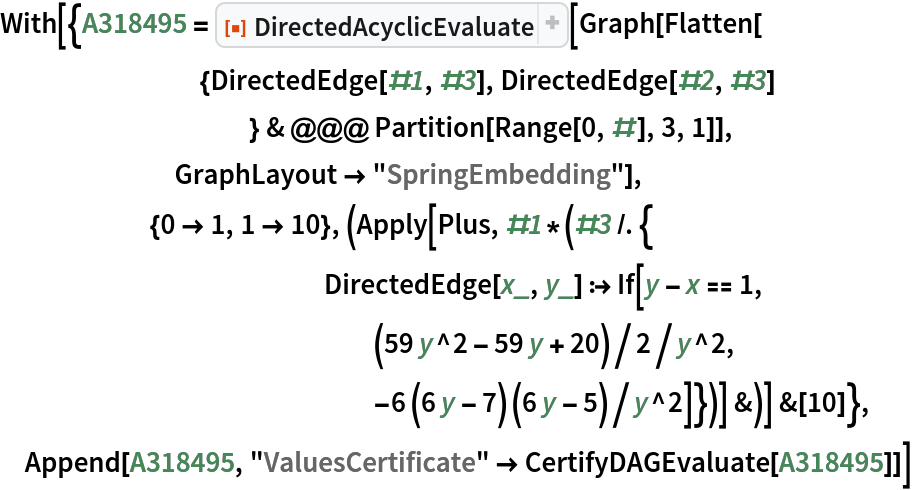Out=### Possible Issues (3)

Invalid inputs result in a failure message:

 In:=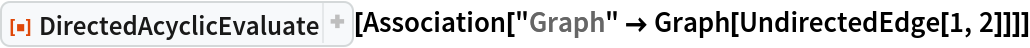Out=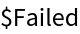In:=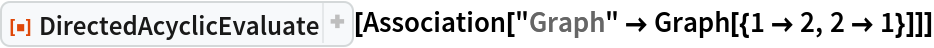Out=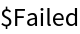MultiwayEvaluate ignores double edges:

 In:=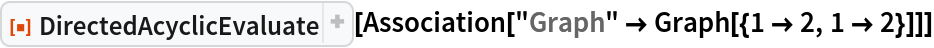Out=If necessary, a corrected input could assign factor of 2 via EdgeWeights:

 In:=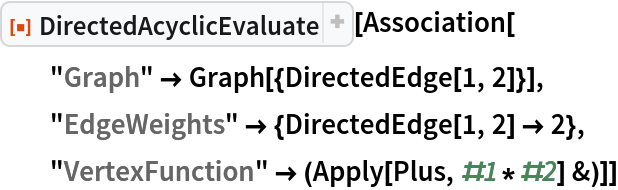Out=### Neat Examples (4)

Calculate a Fourier transform with only n Log2 n complexity (n=32):

 In:=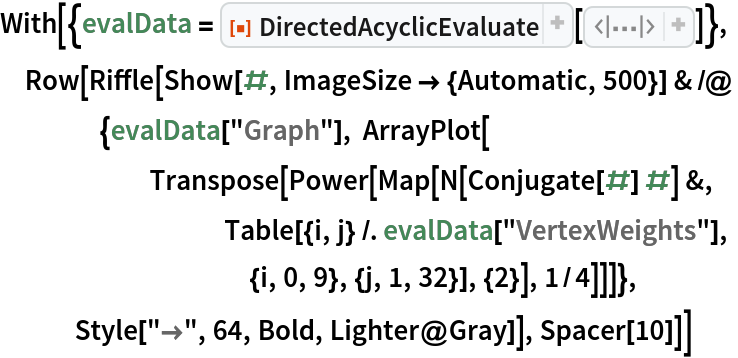Out=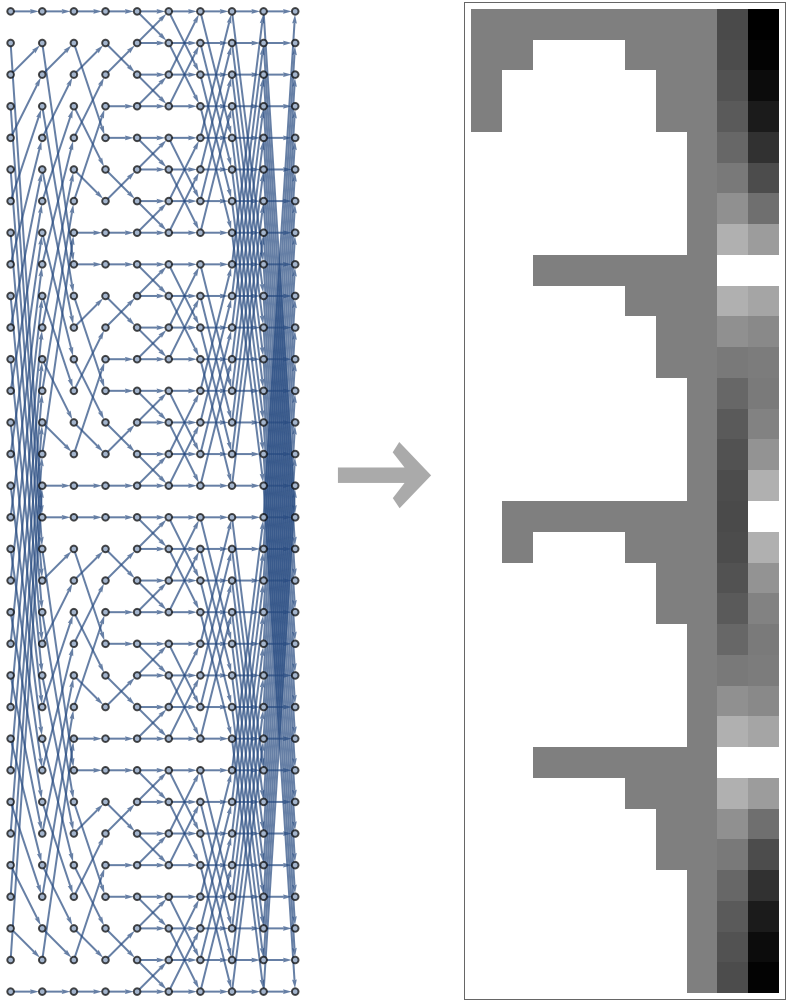Calculate the first few Fubini numbers (OEIS A000670):

 In:=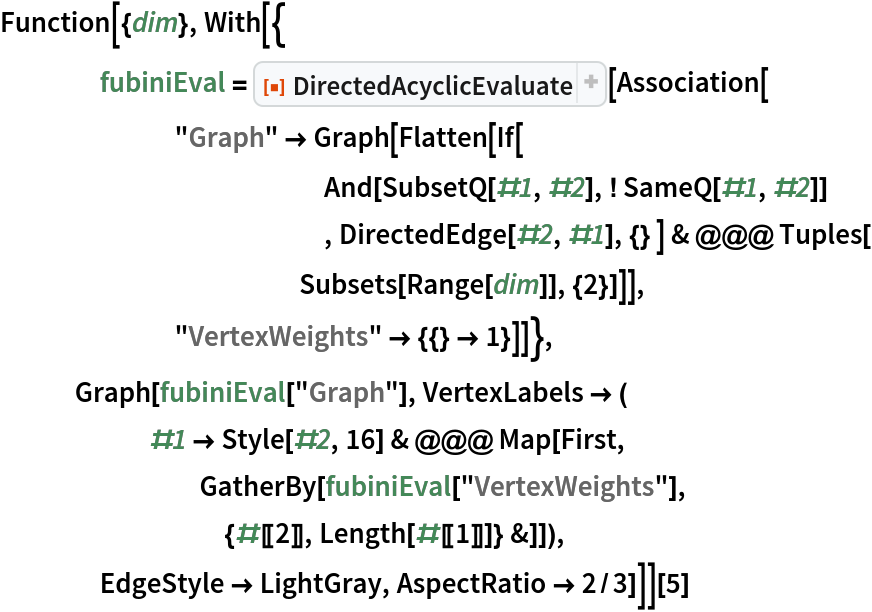Out=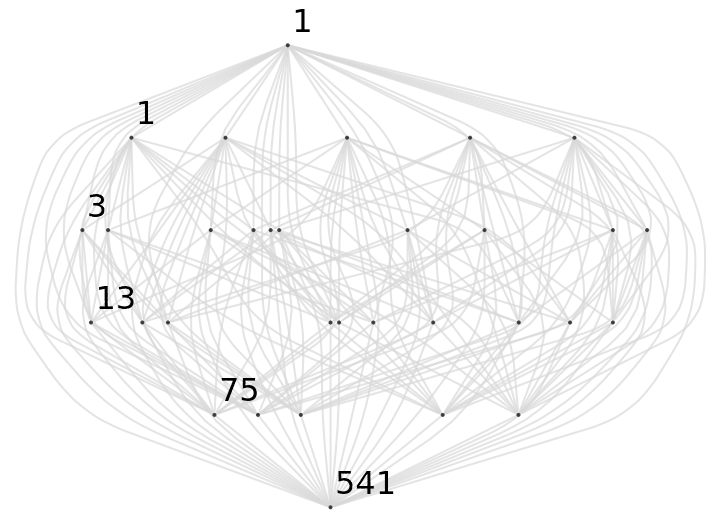Count corner-to-corner walks on three-dimensional grids:

 In:=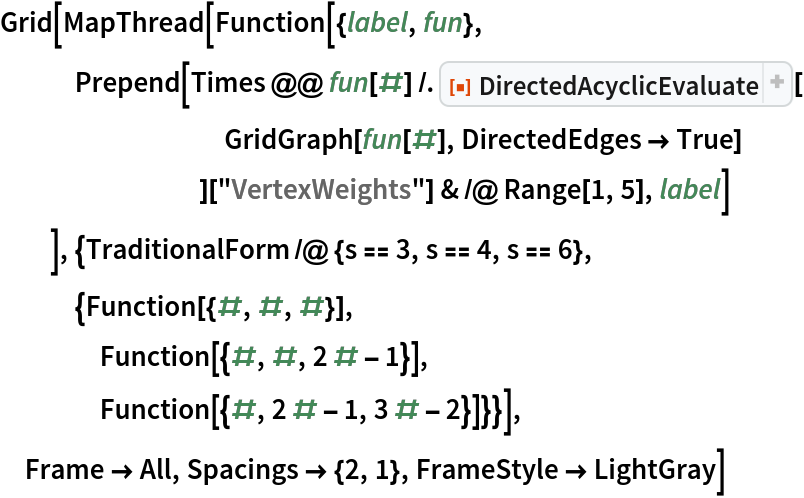Out=Compare with series expansion of Ramanujan's elliptic integrals:

 In:=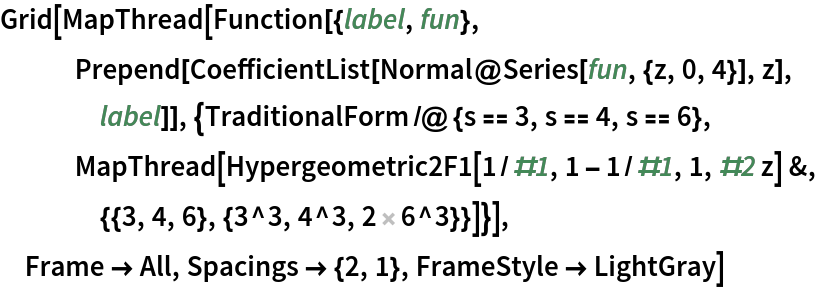Out=Count corner-to-corner walks on the cells of MengerMesh when n=2:

 In:=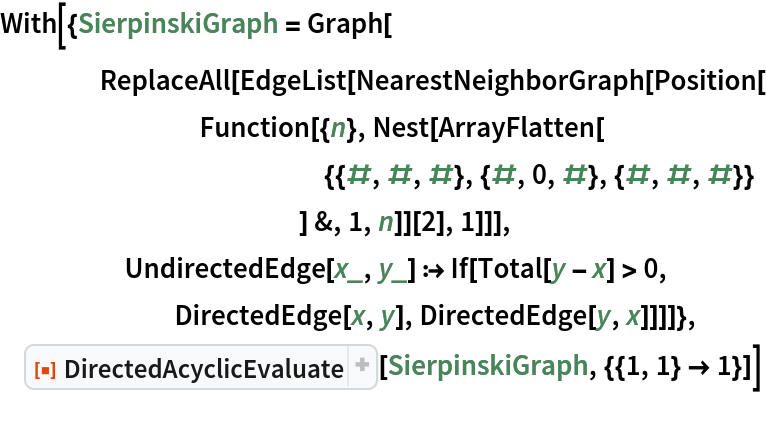Out=Repeat this calculation for sequential inputs, then output maximum path counts:

 In:=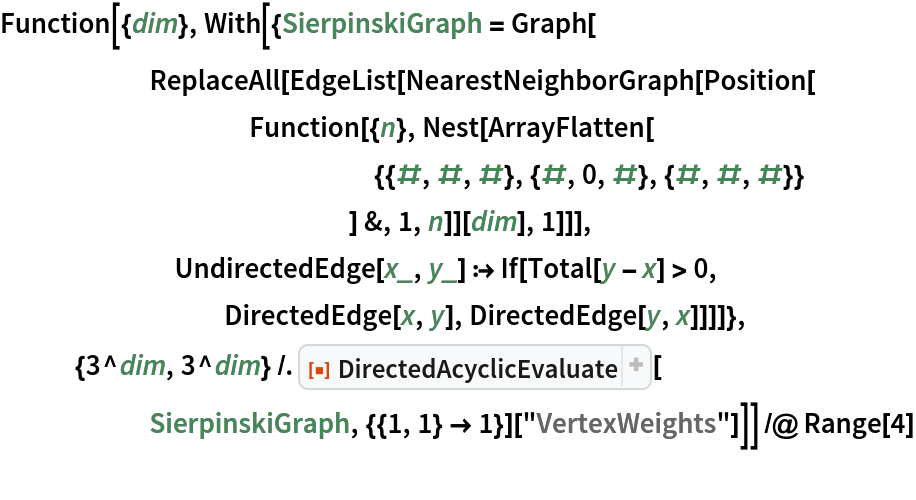Out=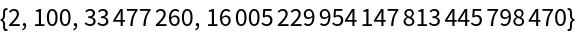Try a similar calculation for the Menger sponge (a.k.a. MengerMesh with d=3):

 In:=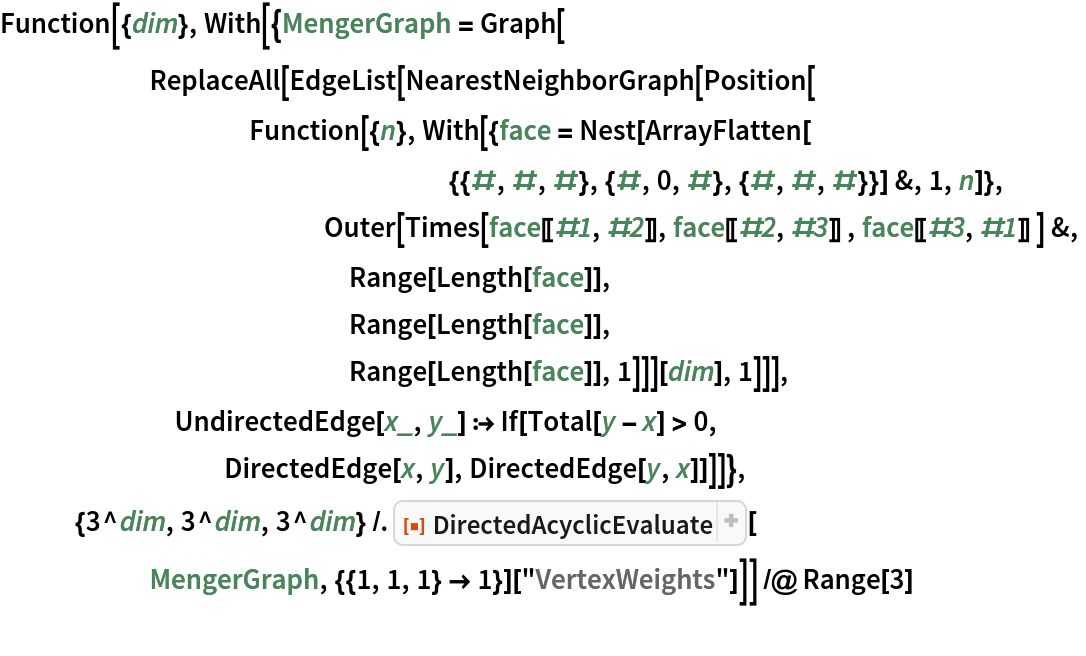Out=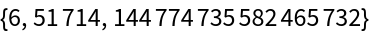## Version History

• 2.0.0 – 08 November 2022
• 1.1.0 – 03 May 2022
• 1.0.0 – 11 April 2022

## Author Notes

Significant revisions to improve Vertex Function + Rename + more better examples (also fixed typo).

Optimized handling of EdgeWeights